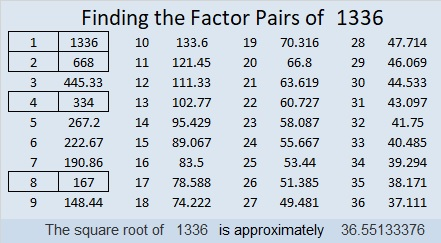# 1336 and Level 3

To solve this level 3 puzzle, start with the clue at the top of the grid, write the factors from 1 to 10 in the appropriate places, then do the same for each factor going down the puzzle row by row.Print the puzzles or type the solution in this excel file: 12 factors 1333-1341

Here’s some information about the puzzle number, 1336:

• 1336 is a composite number.
• Prime factorization: 1336 = 2 × 2 × 2 × 167, which can be written 1336 = 2³ × 167
• 1336 has at least one exponent greater than 1 in its prime factorization so √1336 can be simplified. Taking the factor pair from the factor pair table below with the largest square number factor, we get √1336 = (√4)(√334) = 2√334
• The exponents in the prime factorization are 3 and 1. Adding one to each and multiplying we get (3 + 1)(1 + 1) = 4 × 2 = 8. Therefore 1336 has exactly 8 factors.
• The factors of 1336 are outlined with their factor pairs in the graphic below.1336 is the difference of two squares two ways:
335² – 333² = 1336
169² – 165² = 1336

This site uses Akismet to reduce spam. Learn how your comment data is processed.